# Bomb Lab Write-up

In this write-up, I will show you how i solve bomb lab challenge.
First bomb lab is a Reverse Engineering challenge, you have to read its assembly to find the message that expected by program.

-ida free

## Starting challenge

First we will face the main function:## Phase_1: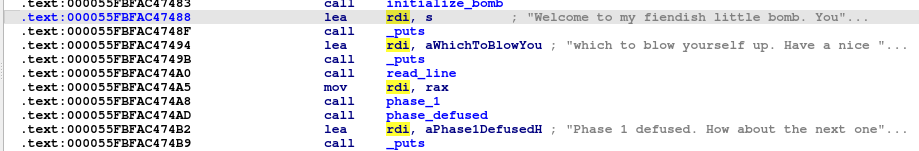First, the program prints these strings “Welcome to my fiendish little bomb. You” and “which to blow yourself up. Have a nice “ Then, we will find a calling of read_line function which take an input from user, and then mov this input to rdi as an argument to function phase1, then calling this functionIn internal code of phase 1 function, we will find that the function copy string “I am just a renegade hockey mom.” into rsi register, and then calling strings_not_equal function, function take the user input and the value in rsi and if they are the same it will return 0, and then test xor the eax if it not equal zero “which means that strings not the same”, it will jump to this location loc_55FBFAC475C4 which will call explode_bomb function, and if it equal zero the function will return to main and calling phase_defused, and print this string “Phase 1 defused. How about the next one”, and here we completed this phase.

## Phase_2: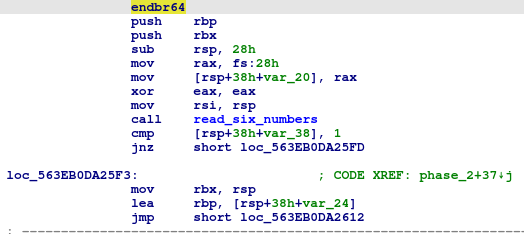In this phase, we will find some function initialization firstly and clear the eax register, and we will see a calling for function read_six_number which tells us how our input format should be. And then, we will see a comparison between a number and 1, and if this number doesn’t equal 1, then a bomb will be exploded, so we can make sure that our first number is 1.

So, now we will jump to loc_55901F1135F3 which the program will prepare to loop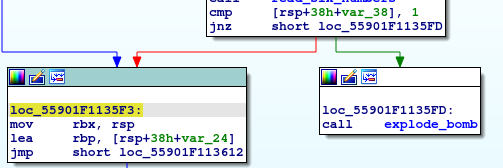In this location we will see that moving a value in rbx to eax “we notice that that the value of rbx is 1”, then we will find that the program adding eax to itself and repeat it.
So if we if we keep tracing this loop with 6 iterations “read_six_numbers”, We will figure out that our right sequence should be 1 2 4 8 16 32, and here we completed this phase.

## Phase_3:In this phase, we will find some function initialization firstly and clear the eax register, and then we will see a loading of two intergers in rsi, then calling ___isoc99_sscanf which check the number of inputs and return this number as an output, so if we see the content of eax we will see that it contains 2.If we continue with the asm we will find that there is checking on the first input with 7 so we can figure out that there are 7 switch cases in this phase, and if the first input is greater than 7 it will call explode_bomb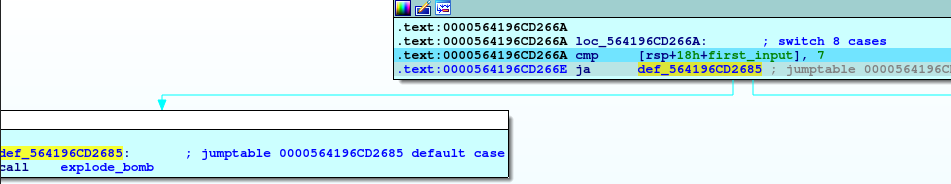In this section, if we move with asm step by step we will figure out that these instructions move the location of case 4 into rax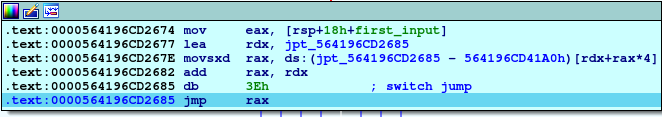Jumping to case 4:In this section, we will see that there is an adding and subtracting with the same value with eaxIf we view the content of eax we will see that it will be (0)After these operations we will see that the program compare the second input we typed it with (0) and if this input not equal, then explode_bomb will be called

So, we can conclude that the second input for 4 is 0

Password: 4 0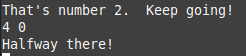“note that we have 7 cases so we have another passwords”

## Phase_4:

In this phase also we will as usuall some function intailization, and then we will find calling an ___isoc99_sscanf function and comparing the eax which contain the output or return from this function with 2 and if not equal will call explode_bomb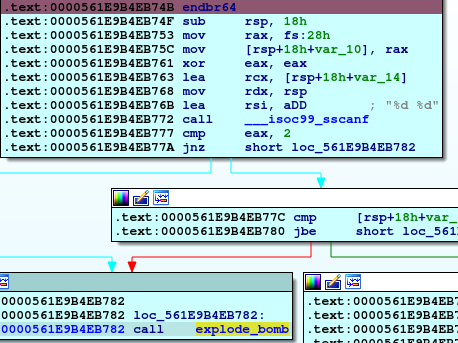So, the first information we founded is that we must enter 2 inputs.

So, we enter 2 inputs and keep going in the code, we will find another comparing , at this time between first input and 0Eh (14 in decimal) and jumping if below or equal so our first input should not be greater than 14.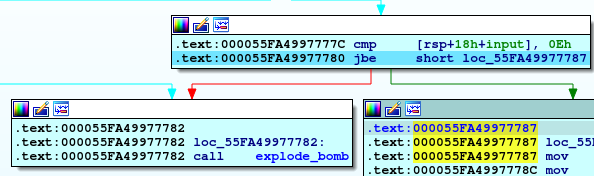So, if our first input is less than or equal 14, we will move to another piece of code which move 0Eh to edx and 0 to esi, our first input to edi, and then calling func4 and compare the return value from it with 0Ah (10 in decimal) if not equal will explode the bomb so let’s walk through func4.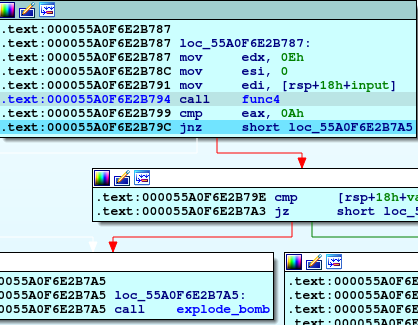If we look inside this function we will see some math inside.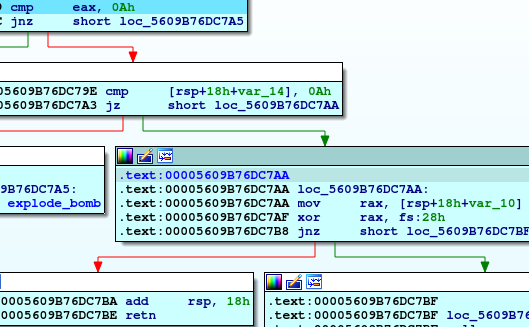So we can conclude from the rest of code that the second input shoud be 0Ah (10 in decimal), and the first input should be the number that if we pass it as a parameter to func4 will produce 10, so if we keep tracking this math we will find that the first input should be 3.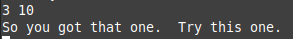Password: 3 10

## Phase_5:

So, in this phase our first as usual, we will see fuction intailization and checking the number of inputs by ___isoc99_sscanf which expected to be 2 or explode_bomb will be called.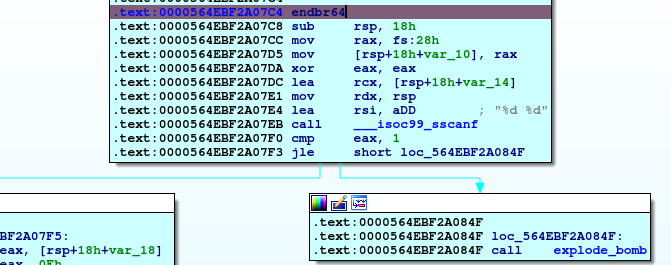So, now we see some logic.
First, moving the first input to eax, doing AND operation with 0Fh (15 in decimal) and then moving the result again to first input location, then comparing this result with 0Fh and if equal, calling explode_bomb.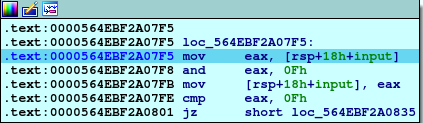So, the first thing to be noticed that our first input should not be 15.

Now we see that ecx and edx contain 0, and rsi point to first element in array, so with this structure we can figure out that there is looping on this array. ![](/assets/images/reverse-engineering/bomb-lab/phase5/loop_structure.png)
So, in this loop we loop on array and adding each element to **ecx** until the value be 0Fh, and then if we meet this condition we will out of loop.

After that, we will see moving 0Fh to our first input location and checking the edx if equal 0Fh (meaning looping on all element in array because edx used as counter) if not explode_bomb will be called.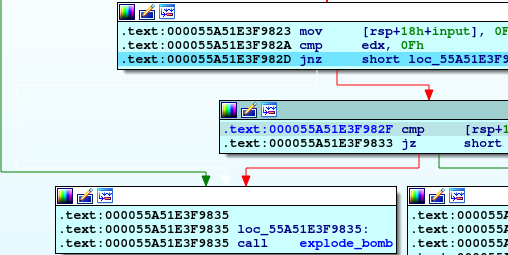Actually i get stuck in this loop so i try to enter all number from 0 to 14 (which very bad technique to follow), and figure out that 5 meet the condition and then we can easily find the next input by comparing the ecx so the next input will be 115.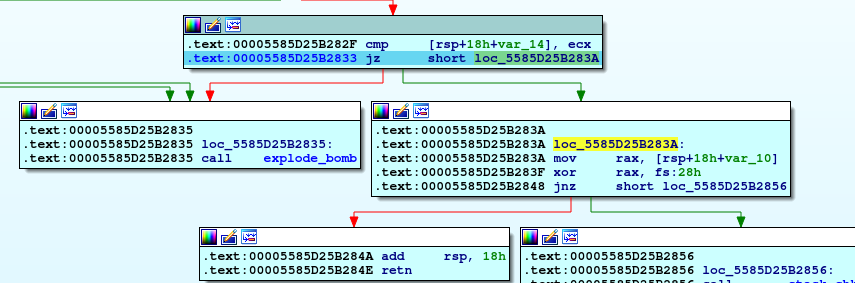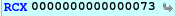Password: 5 115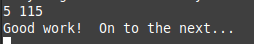## Phase_6:So, now again with function intailization, and calling read_six_numbers function so, first thing to notice that we need to enter six inputs in this phase.

If you take a look on the code you will notice that there are three loops.First loop take every element in array and subtract 1 from it and check if above 5, if true, then explode_bomb will be called.
So, second thing to notice that all six number should be less that or equal 6.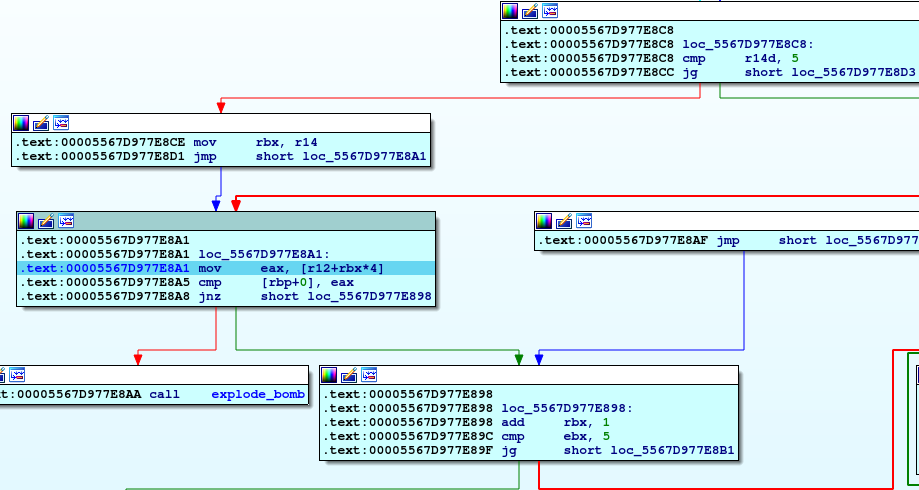This loop take above element we checked it and compare it by all numbers in the input series to make sure that there is no duplication between inputs numbers.

So, now we have six numbers, all numbers are less than or equal 6, and no duplication between them.

You can imagine these loops like this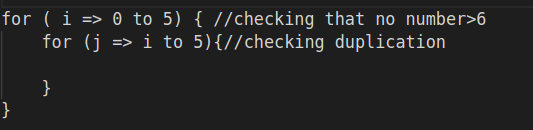So, our numbers are (1 2 3 4 5 6), but it’s not the correct password for the phase, so let’s keep going.I didn’t understand actually what this loop do, but if you notice that there is a something called node1, if you investigate it you will find all numbers from 1 to 6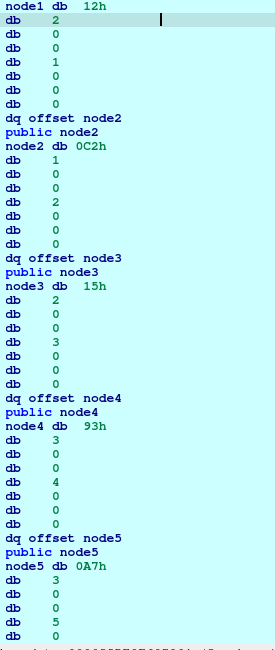I search on internet about meaning of these structures and i found that our six numbers are labels and 1C2h, 215H, etc are values and our password should be ordered by these values descendingly.
So now we can get our password.

Password: 5 4 3 1 6 2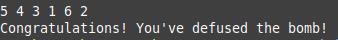Now we finished this challenge, but we have a secret phase will be solved later.

Categories:

Updated: